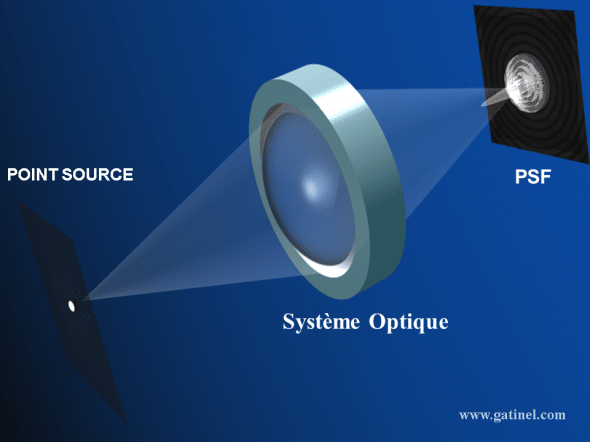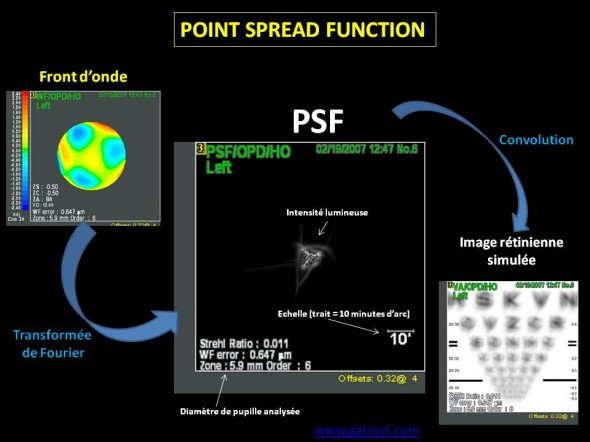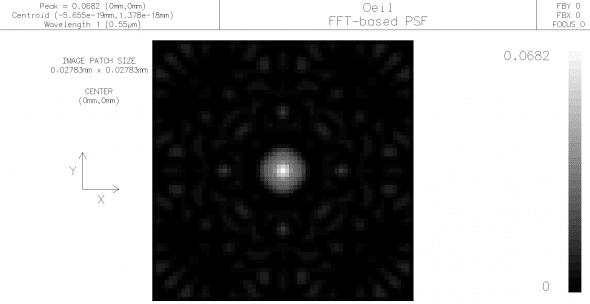+ +

# PSF - Point Spread Function

#### General information

Acronym for Point Spread Function (the Point spread function). The PSF represents the spatial distribution of the light intensity in the image of an optical system, formed from a point source object plane. More the image formed from the point source (PSF) is ad hoc form, the optical system is "faithful". Even for a 'perfect' optics, devoid of optical aberrations, the PSF is not a point pais a disk called Airy task, due to the effects of diffraction by the edges of the opening of the light waves. Depending on the light source (mono or polychromatic), the PSF can be calculated for a wavelength (monochromatic) or a set of (polychromatic) wavelength.The image of a point source formed by an optical system in the image plane is called "Point Spread Function" (the point spread function). The image is affected by diffraction:

#### Calculation and representation of the PSF of the eye

For a healthy eye, optical aberrations and diffraction are responsible for a deterioration of the PSF. Optical aberrations are responsible for the degradation of the image for greater than 2 mm pupil diameter. When the diameter of the pupil is less than 2 mm, the diffraction of the edges of the pupil is at the root of sprawl bright.

The aberrometres calculated the ocular PSF from the collection of the wave front, through a transformed of Fourier. The transformed of Fourier wave gives function the PSF in amplitude, which the square of the module provides the PSF in intensity.  The convolution operation allows to simulate the final image of an object likened to a set of source points: each source point is convoluted with the PSF in intensity.Calculation of the PSF from the wave front and representation. The simulated retinal image is obtained by convolution.

The point spread function is therefore a fundamental parameter in the evaluation of any optical imaging system, and provides a direct estimate of the retinal image quality in ophthalmology.

In the absence of significant light diffusion, the PSF is directly related to the function of aberration of the Wavefront at the level the pupillary opening through a transformed of Fourier. In case increased light distribution (loss of transparency of the tissue: cataract, corneal haze, etc.), the appearance of the PSF can be degraded: the OPD by double passage (OQAS) provides a representation of the more functional PSF

#### PSF and light intensity

The function that describes the way in which an imaging system transforms a point object when it transfers the plan object to an image plane can be described as function of spreading of the point (FEP), the most commonly used English equivalent is the "Point Spread Function" (PSF). The eye PSF is the function that describes the spatial distribution in intensity of the light distribution of the retinal image of an object point. It combines the effect both of diffraction and aberrations. It can also be understood as the function that describes the probability of photon distribution in the image plane which serainet issued by the point source.

In case of reduction of transparency of one or more ocular elements, the PSF also is affected by the dissemination.  Because of the various optical imperfections, the image will be more spread out and wider than an idealized object point.

#### PSF and image of the source object, convolution

Any object can be represented as a set of point sources, each of which is imaged as a PSF by the optics of the eye. Most of the PSF is dense and symmetrical, the more the fidelity of the image. When dealing with an object such as a two-dimensional set of points of various intensity, the PSF determines how the intensity of each object point is converted into intensity at each image point. The operation that is conceptually the translation a point of an object to the level of the retinal image (taking into account the magnification, diffraction and sprawl of aberrations by the exit pupil of the eye) named after convolution operation. More the OGP is important, the more overlap between the points of the irradiation of images profile, therefore reducing the resolution of the real points.

Thus, the image is the result of Assembly (convolution) of each of these PSF.

#### Representation of the PSF

. The representation of the PSF is usually done in white on black background (intensity), or figure an angular distance scale which specifies the extension of the PSF. Recall that in the case of an eye perfectly corrected for all optical aberrations, the PSF would take the appearance of a drive of Airy (diffraction effect), the angular diameter (from the Center to the first 'light extinction') is equal to: 2.44 x wavelength / pupillary diameter).

The Strehl ratio is an indicator of the 'quality' of the measured PSF. He cortrespond to the relationship between the height of the peak of the measured PSF and that of the 'ideal' PSF (Airy task). The criterion of Marshal stipulates that when the Strehl ratio is greater than 0.8, the optical system can be considered as only "limited by diffraction.

The human eye is far from possessing such optical quality: a strehl ratio greater than 0.1 represents an above average optical performance. Here is a representation of light spread of a model eye (Arizona Eye Model), in monochromatic terms (0.55 microns):Theoretical representation of the light spreading from a point source on the retina of a theoretical look (Arizona Eye Model). The Strehl ratio is equal to 0.0682 (pupil of 4.4 mm), due to aberrations optiques.de high degree caused by the imperfect (opposite of an optimal stigma) asphericity of ocular surfaces.